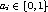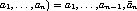## 160. Topology Inquiry FunctionsUp: Topology Constructors Next: Cartesian Shift Coordinates Previous: General (Graph) Constructor

If a topology has been defined with one of the above functions, then the topology information can be looked up using inquiry functions. They all are local calls.

 MPI_TOPO_TEST(comm, status) IN comm communicator (handle) OUT status topology type of communicator comm (state)

int MPI_Topo_test(MPI_Comm comm, int *status)

MPI_TOPO_TEST(COMM, STATUS, IERROR)
INTEGER COMM, STATUS, IERROR
int MPI::Comm::Get_topology() const
The function MPI_TOPO_TEST returns the type of topology that is assigned to a communicator.

The output value status is one of the following:
MPI_GRAPH graph topology
MPI_CART Cartesian topology
MPI_UNDEFINED no topology

 MPI_GRAPHDIMS_GET(comm, nnodes, nedges) IN comm communicator for group with graph structure (handle) OUT nnodes number of nodes in graph (integer) (same as number of processes in the group) OUT nedges number of edges in graph (integer)

int MPI_Graphdims_get(MPI_Comm comm, int *nnodes, int *nedges)

MPI_GRAPHDIMS_GET(COMM, NNODES, NEDGES, IERROR)
INTEGER COMM, NNODES, NEDGES, IERROR
void MPI::Graphcomm::Get_dims(int nnodes[], int nedges[]) const
Functions MPI_GRAPHDIMS_GET and MPI_GRAPH_GET retrieve the graph-topology information that was associated with a communicator by MPI_GRAPH_CREATE.

The information provided by MPI_GRAPHDIMS_GET can be used to dimension the vectors index and edges correctly for the following call to MPI_GRAPH_GET.

 MPI_GRAPH_GET(comm, maxindex, maxedges, index, edges) IN comm communicator with graph structure (handle) IN maxindex length of vector index in the calling program (integer) IN maxedges length of vector edges in the calling program (integer) OUT index array of integers containing the graph structure (for details see the definition of MPI_GRAPH_CREATE) OUT edges array of integers containing the graph structure

int MPI_Graph_get(MPI_Comm comm, int maxindex, int maxedges, int *index, int *edges)

MPI_GRAPH_GET(COMM, MAXINDEX, MAXEDGES, INDEX, EDGES, IERROR)
INTEGER COMM, MAXINDEX, MAXEDGES, INDEX(*), EDGES(*), IERROR
void MPI::Graphcomm::Get_topo(int maxindex, int maxedges, int index[], int edges[]) const
 MPI_CARTDIM_GET(comm, ndims) IN comm communicator with Cartesian structure (handle) OUT ndims number of dimensions of the Cartesian structure (integer)

int MPI_Cartdim_get(MPI_Comm comm, int *ndims)

MPI_CARTDIM_GET(COMM, NDIMS, IERROR)
INTEGER COMM, NDIMS, IERROR
int MPI::Cartcomm::Get_dim() const
The functions MPI_CARTDIM_GET and MPI_CART_GET return the Cartesian topology information that was associated with a communicator by MPI_CART_CREATE. If comm is associated with a zero-dimensional Cartesian topology, MPI_CARTDIM_GET returns ndims=0 and MPI_CART_GET will keep all output arguments unchanged.
 MPI_CART_GET(comm, maxdims, dims, periods, coords) IN comm communicator with Cartesian structure (handle) IN maxdims length of vectors dims, periods, and coords in the calling program (integer) OUT dims number of processes for each Cartesian dimension (array of integer) OUT periods periodicity ( true/ false) for each Cartesian dimension (array of logical) OUT coords coordinates of calling process in Cartesian structure (array of integer)

int MPI_Cart_get(MPI_Comm comm, int maxdims, int *dims, int *periods, int *coords)

MPI_CART_GET(COMM, MAXDIMS, DIMS, PERIODS, COORDS, IERROR)
INTEGER COMM, MAXDIMS, DIMS(*), COORDS(*), IERROR
LOGICAL PERIODS(*)
void MPI::Cartcomm::Get_topo(int maxdims, int dims[], bool periods[], int coords[]) const

 MPI_CART_RANK(comm, coords, rank) IN comm communicator with Cartesian structure (handle) IN coords integer array (of size ndims) specifying the Cartesian coordinates of a process OUT rank rank of specified process (integer)

int MPI_Cart_rank(MPI_Comm comm, int *coords, int *rank)

MPI_CART_RANK(COMM, COORDS, RANK, IERROR)
INTEGER COMM, COORDS(*), RANK, IERROR
int MPI::Cartcomm::Get_cart_rank(const int coords[]) const
For a process group with Cartesian structure, the function MPI_CART_RANK translates the logical process coordinates to process ranks as they are used by the point-to-point routines.

For dimension i with periods(i) = true, if the coordinate, coords(i), is out of range, that is, coords(i) < 0 or coords(i)dims(i), it is shifted back to the interval
0coords(i) < dims(i) automatically. Out-of-range coordinates are erroneous for non-periodic dimensions.

If comm is associated with a zero-dimensional Cartesian topology, coord is not significant and 0 is returned in rank.

 MPI_CART_COORDS(comm, rank, maxdims, coords) IN comm communicator with Cartesian structure (handle) IN rank rank of a process within group of comm (integer) IN maxdims length of vector coords in the calling program (integer) OUT coords integer array (of size ndims) containing the Cartesian coordinates of specified process (array of integers)

int MPI_Cart_coords(MPI_Comm comm, int rank, int maxdims, int *coords)

MPI_CART_COORDS(COMM, RANK, MAXDIMS, COORDS, IERROR)
INTEGER COMM, RANK, MAXDIMS, COORDS(*), IERROR
void MPI::Cartcomm::Get_coords(int rank, int maxdims, int coords[]) const
The inverse mapping, rank-to-coordinates translation is provided by MPI_CART_COORDS. If comm is associated with a zero-dimensional Cartesian topology, coords will be unchanged.
 MPI_GRAPH_NEIGHBORS_COUNT(comm, rank, nneighbors) IN comm communicator with graph topology (handle) IN rank rank of process in group of comm (integer) OUT nneighbors number of neighbors of specified process (integer)

int MPI_Graph_neighbors_count(MPI_Comm comm, int rank, int *nneighbors)

MPI_GRAPH_NEIGHBORS_COUNT(COMM, RANK, NNEIGHBORS, IERROR)
INTEGER COMM, RANK, NNEIGHBORS, IERROR
int MPI::Graphcomm::Get_neighbors_count(int rank) const
MPI_GRAPH_NEIGHBORS_COUNT and MPI_GRAPH_NEIGHBORS provide adjacency information for a general graph topology.
 MPI_GRAPH_NEIGHBORS(comm, rank, maxneighbors, neighbors) IN comm communicator with graph topology (handle) IN rank rank of process in group of comm (integer) IN maxneighbors size of array neighbors (integer) OUT neighbors ranks of processes that are neighbors to specified process (array of integer)

int MPI_Graph_neighbors(MPI_Comm comm, int rank, int maxneighbors, int *neighbors)

MPI_GRAPH_NEIGHBORS(COMM, RANK, MAXNEIGHBORS, NEIGHBORS, IERROR)
INTEGER COMM, RANK, MAXNEIGHBORS, NEIGHBORS(*), IERROR
void MPI::Graphcomm::Get_neighbors(int rank, int maxneighbors, int neighbors[]) const

Example

Suppose that comm is a communicator with a shuffle-exchange topology. The group has 2n members. Each process is labeled by a1 , ..., an with, and has three neighbors: exchange((), shuffle(a1 , ..., an )= a2 , ..., an, a1, and unshuffle(a1 , ..., an ) = an , a1 , ... , an-1. The graph adjacency list is illustrated below for n=3.

 2|c| node exchange shuffle unshuffle neighbors(1) neighbors(2) neighbors(3) 0 (000) 1 0 0 1 (001) 0 2 4 2 (010) 3 4 1 3 (011) 2 6 5 4 (100) 5 1 2 5 (101) 4 3 6 6 (110) 7 5 3 7 (111) 6 7 7

Suppose that the communicator comm has this topology associated with it. The following code fragment cycles through the three types of neighbors and performs an appropriate permutation for each.

```C  assume: each process has stored a real number A.
C  extract neighborhood information
CALL MPI_COMM_RANK(comm, myrank, ierr)
CALL MPI_GRAPH_NEIGHBORS(comm, myrank, 3, neighbors, ierr)
C  perform exchange permutation
CALL MPI_SENDRECV_REPLACE(A, 1, MPI_REAL, neighbors(1), 0,
+     neighbors(1), 0, comm, status, ierr)
C  perform shuffle permutation
CALL MPI_SENDRECV_REPLACE(A, 1, MPI_REAL, neighbors(2), 0,
+     neighbors(3), 0, comm, status, ierr)
C  perform unshuffle permutation
CALL MPI_SENDRECV_REPLACE(A, 1, MPI_REAL, neighbors(3), 0,
+     neighbors(2), 0, comm, status, ierr)
```Up: Topology Constructors Next: Cartesian Shift Coordinates Previous: General (Graph) Constructor